#Quality Digest

Featured Product
This Week in Quality Digest Live
Statistics Features
Donald J. Wheeler
Using process behavior charts in a clinical setting
Donald J. Wheeler
No one really understands kurtosis. Here’s why.
Alan Metzel
Introducing the Enhanced Perkin Tracker
Donald J. Wheeler
What you think you know may not be so
Tony Boobier
Why data leaders need to master words as well as statistics
Statistics News
New capability delivers deeper productivity insights to help manufacturers meet labor challenges
Day and a half workshop to learn, retain, and transfer GD&T knowledge across an organization
Elsmar Cove is a leading forum for quality and standards compliance
InfinityQS’ quality solutions have helped cold food and beverage manufacturers around the world optimize quality and safety
User friendly graphical user interface makes the R-based statistical engine easily accessible to anyone
Collect measurements, visual defect information, simple Go/No-Go situations from any online device
Good quality is adding an average of 11 percent to organizations’ revenue growth
Ability to subscribe with single-user minimum, floating license, and no long-term commitment
A guide for practitioners and managersBio

Statistics

## Setting the Process Aim, Part 1

Published: Monday, May 11, 2020 - 12:03

All articles in this series:

Lean production of multiple products is built on the assumption that the process aim can be properly set for each short production run. This article will describe how to set the process aim so that your short production runs can be on target.

In a lean production environment, without a bank of in-process inventory to cushion the impact, and without adequate lead time to allow for reworking or refabricating the product, a single off-target run can shut down an assembly operation and create a massive pile of unintended, in-process inventory. (I once saw 25 jumbo jets parked outside their assembly building. When I asked why they were parked there, I was told that they were all waiting for parts—a billion dollar pile of in-process inventory!)

### Aim vs. consistency

A plant had three suppliers for a piece of wire. When a shipment arrived they collected a sample of five pieces and measured their lengths. These five values were subgrouped together and placed on an average and range chart. The charts for each of the three suppliers, drawn to the same vertical scale, are shown in figure 1.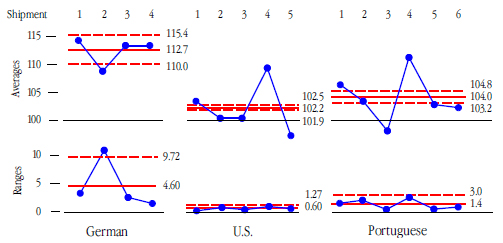Figure 1: Average and range charts for wire lengths

The specification for this piece of wire was 100 mm ± 4 mm. These specifications are shown on the histograms for these three suppliers in figure 2.Figure 2: Histograms for wire lengths

Only one of the 20 pieces from the German supplier was within the specifications! Moreover, the points outside the limits on the average and range chart for the German supplier show inconsistency both between the shipments and within the shipments. Based on this performance, the German supplier was dropped.

The range chart for the U.S. supplier shows great consistency within each shipment, but the average chart shows completely different average lengths between the shipments. The histogram for the U.S. supplier reinforces this interpretation by showing four separate clusters. The first cluster on the right is shipment 5. The next cluster is for shipments 2 and 3. Next comes shipment 1, followed by nonconforming shipment 4. So while the U.S. supplier has great consistency within each shipment, they have a real problem with setting the process aim.

This story repeats itself with the Portuguese supplier. They have good consistency within each shipment but real differences from shipment to shipment. Based on the charts above, the U.S. supplier and Portuguese supplier were told that their problem was setting up each production run on target.

### The process aim, the process average, and the target

Let the “process aim” denote all that is directly manipulated by the operator: those values at which the adjustable process inputs are set. Here we restrict our attention to those inputs that are easily changed, and are therefore part of the “steering wheel” for the process.

Let the “process average” denote the average value of some product characteristic produced while the process is operated at a given process aim. Since this process average is generally unknown, it is commonly estimated by the average of measured samples.

Finally let the “target” denote the desired value for the process average.

In the preceding example the target value was 100 mm. The average for each subgroup approximates the process average for each shipment, and the process aim would consist of those inputs to the wire fabrication process which the operator can manipulate. Thus, the process aim is what you set, the process average is what you get, and the target is what you want.

With this nomenclature, the objective of an aim-setting procedure is to adjust the process aim until the process average is close enough to the target to result in a satisfactory production run.

Why settle for close? Why not make the process average equal to the target? Simply because our estimates of the process average are always subject to sampling variation. This guarantees that no procedure will ever be able to consistently make the process average exactly equal to the target. The best that can ever be achieved in practice is to consistently get the process average close to the target.

In order to do this, any effective aim-setting procedure must use an adequate amount of data in conjunction with an appropriate decision rule. (An inadequate amount of data can be worse than guessing. The wrong decision rule will waste effort through over adjustment or under adjustment.)

It is always a mistake to adjust the process aim before you have a detectable signal that the process average is off target.

### Aim setting vs. process monitoring

Since we will use a process behavior chart as one of our aim-setting procedures, it is important to pause to consider the difference between setting the process aim and monitoring process behavior. As the name implies, when we track the operation of a process with a process behavior chart we are asking the question “Has a change occurred?” Since we do not want to react to false alarms, the process behavior chart is set up to be conservative. We want to be reasonably certain that a change has occurred before we intervene. In line with this conservative approach, decades of practice have taught us that we rarely need anything other than detection rule one—a point outside the three sigma limits—when monitoring for unknown process changes.

However, in the aim-setting mode things are different. When we have deliberately changed the process aim, the question becomes “Has our change had the desired effect?” Here we are no longer trying to prove that a change has occurred. The burden of proof has shifted. We want to know if our deliberate change has gotten the process average close to the target.

To answer this new question we need to increase the sensitivity of our process behavior chart, and the proven way to increase the sensitivity without an undue increase in false alarms is to use the Western Electric zone tests. These detection rules are summarized in the context of setting the process aim below.

Detection Rule One: Three-sigma limits are defined here as [Target ± 3 Sigma(X)]. A single point outside these three-sigma limits is taken as a signal that the process average is detectably different from the target value.

Detection Rule Two: Two-sigma lines are defined here as [Target ± 2 Sigma(X)]. A run of two out of three successive values on the same side of the target value and outside the two-sigma lines is taken as a signal that the process average is detectably different from the target value.

Detection Rule Three: One-sigma lines are defined here as [Target ± Sigma(X)]. A run of four out of five successive values on the same side of the target value and outside the one-sigma lines is taken as a signal that the process average is detectably different from the target value.

Detection Rule Four: A run of eight or more successive values on the same side of the target value is taken as a signal that the process average is detectably different from the target value.

Whenever we detect a signal using any one of these rules it will be appropriate to adjust the process aim. In applying these rules we will have to have a value for Sigma(X) for the characteristic being measured. In this context Sigma(X) is a generic reference to any within-subgroup estimate of the process variation. When working with individual values there are only two formulas for Sigma(X):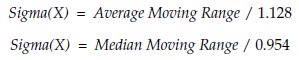If you do not have a value for Sigma(X) for the characteristic being measured you will need to use the second of the two procedures given below.

### Procedure one: The target-centered X chart

So how can we use a process behavior chart to set the process aim? We start off with the assumption that the process aim has been properly set and that the process average is near the target value. We do this by placing the central line of the X chart at the target value. Call this the “target-centered X chart.” (We place the central line at the target value because it is always easier to obtain a contradiction than a confirmation. Moreover, a contradiction provides stronger evidence than does the absence of a contradiction.)

The limits and lines for the target-centered X chart are: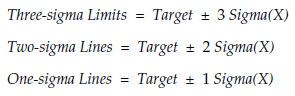As the observations occur, they are plotted on the target-centered X chart. Under these conditions, any point, or any run, that qualifies as a signal using the four detection rules given above may be taken as evidence that the process average is detectably different from the target value, and further adjustments to the process aim are needed.

In making adjustments, the average of the observations collected since the previous adjustment will serve as an estimate of the current value of the process average. After we make an adjustment we will start plotting a new series of observations on the target-centered X chart. We continue in this way until we have 10 successive values with no evidence of the process being off target.

When you shift from aim-setting mode back to process monitoring mode you will want to have a moving range chart. If you wish to include a moving range chart with your target-centered X chart the central line and upper limit may be found using:When using a moving range chart in the aim-setting mode, do not compute a moving range at those points where the process aim is adjusted.

### Example one

Product 16F had a target value of 59.0 units. Process behavior charts provided a Sigma(X) value of 1.80 for this product. With these values the three-sigma limits were 53.6 to 64.4. The two-sigma lines were 55.4 to 62.6. And the one-sigma lines were 57.2 to 60.8.

As the production of Product 16F began the first observation was 61. By itself this value did not indicate any problem with the process aim, so no action was taken. As soon as it was reasonable, a second observation was taken. This value was 66, which signaled the need to adjust the process aim. The average of 61 and 66 is 63.5, so they estimated that the process average needed to be reduced about 4 units. The process aim was adjusted accordingly.Figure 3: Target-centered X chart

The next observed value was 58. No action needed. In fact the next nine values were 61, 61, 58, 56, 59, 58, 57, 62, and 59. As shown in figure 4 this sequence of 10 values contained no signals that the process average was off target. This was taken as an indication that the process average was reasonably close to the target.Figure 4: Target-centered X chart

At this point, as will be explained below, there was better than a 91-percent chance that the process average was within ±1 Sigma(X) of the target value. So they shifted from the aim-setting mode to the process monitoring mode.

### Procedure two: The target-centered XmR chart

But what do we do when we do not have a prior estimate of Sigma(X)? We will have to collect some data to use in estimating Sigma(X). When estimating dispersion we need to use at least 16 moving ranges before our estimate of Sigma(X) will begin to solidify. Estimates of dispersion based on fewer moving ranges will be soft.

However, in the problem of setting the process aim, we rarely will have the luxury of collecting 17 or more observations before we start adjusting the process aim. So, for those cases where a compromise is needed we start with 10 individual values and update our estimate of dispersion when we have 16 or more moving ranges available.

To create a target-centered XmR chart we begin with a central line set equal to the target and plot our observations without the benefit of any limits. We also track the moving ranges for these first 10 values. When we have 10 values plotted on the start-up chart we will compute the average of the nine moving ranges.

Using this average moving range we compute an estimate of Sigma(X) by dividing by d2 = 1.128. With this estimate we can generate the lines needed for the target-centered X chart. That is, we compute the target-centered three-sigma limits, the two-sigma lines, and the one-sigma lines for the X chart. The upper range limit is 3.267 times the average moving range, and the average moving range will be the central line for the range chart. We plot these limits on the start-up chart and check for evidence that the process average is off target.

If the first 10 values signal the need to adjust the process aim we do so and then proceed as we did when a value for Sigma(X) was available.

If the first 10 values fail to signal the need to adjust the process aim, then we continue to collect additional data and plot these values and their moving ranges on the target-centered XmR chart. When 10 additional data are available we recompute the limits and lines using all of the moving ranges. If the new limits show no need to adjust the process aim, decide the process is on target and switch to process monitoring mode.

Since the mR chart is an option with the first procedure, but is a requirement with the second, I shall refer to both procedures collectively as a target-centered XmR chart. The user is expected to understand which procedure is needed from the context.

### Example two

A new process is being set up. The target value for the product is 35. After the initial warm-up period observations are obtained every 2 minutes. The first 10 values are 32, 37, 32, 33, 33, 32, 31, 34, 31, and 32. Figure 5 shows the beginning of the target-centered XmR chart.Figure 5: Initial portion of target-centered XmR chart

The run of eight successive values below the target value of 35 is a signal that the process is not centered on the target. Since the average for these first 10 values was 32.7 the aim was adjusted upward by what was thought to amount to two units.

The average moving range in figure 5 is 1.97. Dividing by d2 = 1.128 we get an estimate of Sigma(X) of 1.75. Using this value we get target-centered three-sigma limits of 29.75 and 40.25; two-sigma lines of 31.5 and 38.5; and one-sigma lines of 33.25 and 36.75. The upper range limit is 6.4.

These lines could be used to evaluate the first 10 observations, but since we already had a signal that this process was off target we simply use these lines to evaluate additional observations from the process as shown in figure 6.Figure 6: Second portion of target-centered XmR chart

The next 10 values show no evidence that the process average is detectably different from the target value of 35. So, as with the target-centered X chart we conclude that the process aim is properly set and switch to using the XmR chart to monitor the process. With 10 values showing no detectable difference between the process average and the target, we can say that the average is within ±1 Sigma(X) of the target with a posterior probability of at least 91 percent.

With 18 moving ranges now available we recompute the average moving range and target-centered three-sigma limits. Here the average moving range is 2.44, resulting in target-centered three-sigma limits of 28.5 to 41.5. The upper range limit becomes 8.0. The revised estimate of Sigma(X) is now 2.16 units. These revised limits are used for monitoring the process.

In this example the process average was approximately one Sigma(X) below the target to start with, and the target-centered XmR chart detected this even before we computed any limits.Figure 7: Third portion of target-centered XmR chart

### Interpretation

So, when we have 10 successive values on the target-centered XmR chart with no evidence of being off target after using all four of the Western Electric zone tests, what can we say about the process average? When we decide to cease to adjust the process aim, how close to the target is the process average? Figure 8 shows how the target-centered XmR chart works in practice.

Assuming that the process average is initially found to be within 12 Sigma(X) on either side of the target, with the more remote values being less likely, we end up with a prior distribution like that shown at the top of figure 8.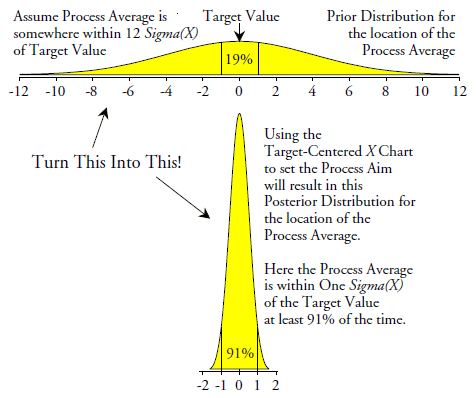Figure 8: How the target-centered X chart works

When we have used the target-centered XmR chart and finally collected 10 successive values with no evidence of being off target we will have turned the prior distribution at the top of figure 8 into the posterior distribution at the bottom. So, while initially we had about a 19-percent chance of being within ± one Sigma(X) of the target, after setting the aim we have at least a 91-percent chance of being within ± one Sigma(X) of the target.

Figure 9 summarizes the posterior distribution above by listing the probabilities that the process average is within selected distances from the target.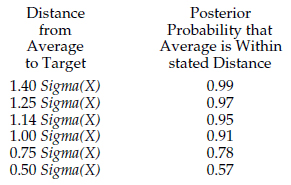Figure 9: How close to the target is the process average

For example, using a target-centered XmR chart to set the process aim will give you a 95 percent upper bound on the average-to-target distance of no more than 1.14 Sigma(X). The average-to-target distance is virtually certain to be less than 1.40 Sigma(X), and it will be less than 1.0 Sigma(X) at least 9 times out of 10. (For more about prior and posterior distributions see appendix one.)

### The economic impact

To assess the economic impact of using the target-centered XmR chart to set the process aim we need to consider the effects of operating when the process average is not exactly equal to the target value. To do this we combine the posterior distribution in figure 8 with the concept of the effective cost of production as outlined in appendix two.

Once we have computed the average effective cost of production that results from using our aim-setting plan, we can compare it with the minimum possible effective cost of production. Since the minimum effective cost of production will occur when the process average is exactly on target, the ratio of the average cost to the minimum cost will characterize the economic impact of our aim-setting procedure. A curve showing these ratios as a function of the process capability is given in figure 10 for the case where all nonconforming product is reworked. In addition there is a second curve which shows the ratios of the average effective cost of use to the minimum effective cost of use.

There we see that using the target-centered XmR chart will result in an average effective cost of production that will always be within 5.6 percent of the minimum possible. Moreover, the average effective cost of use will always stay within 2 percent of the minimum possible.Figure 10: Economic impact with reworked product

So, when all nonconforming product can be reworked, the use of the target-centered XmR chart will get the average effective cost of production within 5.6 percent of the minimum possible effective cost of production regardless of the capability ratio for the process.Figure 11: Economic impact with scrapped product

However, at the other extreme, when all nonconforming product is scrapped, the posterior distribution in figure 8 will result in an average effective cost of production that is within 5 percent of the minimum possible only when the capability ratio exceeds 0.70. To get the average effective cost of production within 3 percent of the minimum you will need to have a capability ratio of 0.80 or greater.

Using a target-centered XmR chart to set the process aim when the capability ratio is less than 0.70 and nonconforming product is scrapped will result in an average cost of production that will be more than 105 percent of the minimum possible effective cost of production. Thus, figure 11 defines the zone where the target-centered XmR chart will no longer be adequate as an aim-setting procedure. Here we will need an aim-setting procedure with a greater ability to get the process on target. Such procedures will be covered in part two.

### Summary

The target-centered XmR chart may be used to get the average-to-target distance to be less than 1.0 Sigma(X) at least 91 percent of the time. This will result in good process economics whenever the process capability ratio exceeds 0.70 or 0.80. When nonconforming product is reworked rather than scrapped the target-centered XmR chart may also be satisfactory with smaller process capabilities.

A target-centered XmR chart may be used as an effective aim-setting procedure that is easily changed into a continuing process monitor when needed. This eliminates the need for multiple procedures and is easy to use in production environments. In aim-setting mode we use the Western Electric zone tests to boost the sensitivity of the chart since we do not have to prove that we have made a change when we adjust the process aim.

When we do not have an estimate of Sigma(X) available we may use the target-centered XmR chart to first obtain a provisional estimate of Sigma(X), and then to update this estimate as more data become available.

Alternative aim-setting techniques that may be used to reliably get the process average closer to the target will be covered in part two.

### Appendix one: Explanation of figure 8

The posterior distribution for the average-to-target distance given at the bottom of figure 8 is the distribution that gives the answers we want to know. It gives the probabilities that a state of nature exists (the process is on target) given a specific observed outcome (the X chart shows no evidence that we are off target after collecting 10 values). Thus, the posterior distribution tells us what we need to know in order to interpret a specific observed outcome.

Probability theory gives us conditional distributions. These are the opposite of the above since they give us the probability of a specific outcome (the X chart shows no evidence that we are off target after collecting 10 values) given that a certain state of nature exists (the average-to-target distance takes on a specific value). Here the conditional distribution comes from the tables of the power function for an XmR chart in reference .

To turn a conditional distribution around to obtain a posterior distribution we have to use a prior distribution for the average-to-target distances. A prior distribution assigns a probability to each value for the average-to-target distances (prior to the process aim being adjusted).

By using the prior distribution with the conditional distribution according to the laws of probability theory (Bayes’ theorem) we can obtain the posterior distribution, and it is the posterior distribution that answers the important question of what is the probability of a state of nature given a specific observed outcome.

The prior distribution shown in figure 8 is sufficiently conservative to give reasonably stable results for the posterior distribution. For example, using a prior that allows the average-to-target distance to range between ±6 Sigma(X) would be only half as wide as the one in figure 8. Yet with this less conservative prior the posterior probability that the average-to-target distance is less than 1.0 Sigma(X) would only be 92 percent vs. the 91 percent found with the more conservative prior. So, by using a sufficiently conservative prior distribution we can usually obtain a reasonably stable posterior distribution that will provide a way to interpret our observed outcomes.

### Appendix two: Explanation of figures 10 and 11

The process capability ratio, Cp, and the centered capability ratio, Cpk, will define an effective cost of production (and also an effective cost of use) for the products produced by your process. The details of this relationship are fully explained in references  and  and are used in references  and .

When Cpk is equal to Cp the effective cost of production will be as small as possible for that value of Cp. This will be the minimum possible effective cost of production for that capability. Here the average-to-target distance will be zero.

As the average-to-target distance increases the value of Cpk will drop relative to Cp , and for each combination of the capability ratios there will be a different effective cost of production.

The posterior distribution defines a probability for each average-to-target distance. Thus, when we hold the value for Cp constant, these probabilities may be used across all the values of Cpk to compute an average effective cost of production for that capability.

Once we have an average effective cost of production, we can compare it with the minimum possible effective cost of production for that capability. These ratios of the average effective cost of production to the minimum effective cost of production will serve to characterize the economic consequences of using the target-centered XmR chart to set the process aim.

In addition to the effective cost of production, the above exercise can also be carried out with the effective cost of use to characterize the impact of the target-centered XmR chart upon using the conforming product. These two sets of ratios are plotted in figures 10 and 11.

References

1. “Tables of the Power Function for Process Behavior Charts,” Donald J. Wheeler and Rip Stauffer, available as a download at www.spcpress.com/pdf/DJW321.pdf

2. Reducing Production Costs, Donald J. Wheeler, SPC Press, Knoxville, Tennessee, 2010.

3. “The Effective Cost of Production and Use,” Quality Digest Daily, Aug. 2, 2010.

4. “The Gaps Between Performance and Potential,” Quality Digest Daily, Sept. 1, 2010.

5. “What is the Economic Zone of Production,” Quality Digest Daily, Oct. 4, 2010.

### Discuss### Donald J. Wheeler

Find out about Dr. Wheeler’s virtual seminars for 2023 at www.spcpress.com. Dr. Wheeler is a fellow of both the American Statistical Association and the American Society for Quality who has taught more than 1,000 seminars in 17 countries on six continents. He welcomes your questions; you can contact him at djwheeler@spcpress.com.